# Scientific Computing with Python¶

A gentle introduction to some elements of scientific programming in Python.

Programming Bootcamp for biology graduate students, September 2013.

Mickey Atwal, Cold Spring Harbor Laboratory. (Lab website)

INTERNET RESOURCES

In Python, dividing an integer by an integer gives you just an integer, removing the remainder. This is a legacy from older programming languages like C. In the future, this feature will change in Python, giving you a real result. If you want it now, you can import the new feature from the future to indicate that '/' means true division.

In :
from __future__ import division


The following are the standard ways of loading some useful Python packages for scientific computing

In :
# load NumPy package and abbreviate the name to 'np'
import numpy as np

# load SciPy package and abbreviate the name to 'sp'
import scipy as sp

# load Matplotlib plotting package and abbreviate the name to 'plt'
import matplotlib.pyplot as plt


We will be working with the text file containing the nucleotide counts of the E. Coli DNA binding sites of the transcription factor CRP (cAMP receptor protein) also known as CAP (catabolite gene activator protein). You can find a copy at http://atwallab.cshl.edu/links/crp_counts_matrix.txt. I will go through the steps of how to download a text file from the web, save it, and open it again in a numerical array format.

In :
# load the module that deals with URL stuff
import urllib

# web URL address of the file

# name of the file to be saved into
filename="data.txt"

# downloads the file and saves it into the local directory
urllib.urlretrieve(url,filename)

Out:
('data.txt', <httplib.HTTPMessage instance at 0x10a435f38>)

This is a small tab-delimited text file where the counts data at each of the 42 nucleotide positions is stored as a series of strings. Let's take a look using the Unix command "cat"

In :
!cat data.txt

109	61	57	115
95	74	62	111
111	70	70	91
89	68	83	102
99	79	72	92
93	66	72	111
83	79	77	103
91	85	70	96
121	63	71	87
143	38	57	104
162	25	38	117
155	28	43	116
127	43	46	126
40	40	29	233
32	16	240	54
30	36	27	249
29	18	254	41
227	41	46	28
74	54	53	161
68	106	77	91
70	81	55	136
131	45	88	78
77	74	100	91
134	60	59	89
32	31	30	249
46	247	14	35
238	29	41	34
60	232	16	34
217	35	45	45
94	62	49	137
116	39	20	167
113	43	24	162
89	51	38	164
100	62	58	122
110	72	67	93
105	62	76	99
108	90	65	79
110	71	72	89
93	76	65	108
102	74	66	100
121	72	44	105
130	49	52	111


We need to convert this to a numerical array of numbers where we can perform computations. The genfromtxt function in NumPy automatically generates a NumPy array from a text file

In :
# loads data from text file and store in an integer NumPy array called 'counts'
counts=np.genfromtxt(filename,dtype=int)


### 2. Working with numerical arrays¶

In :
counts.ndim # what is the dimensionality of the array?

Out:
2
In :
counts.shape # what is the size of the array? (rows, columns)

Out:
(42, 4)
In :
counts.dtype # what is the data type of the array?

Out:
dtype('int64')

#### Array indexing¶

Let's practise some array indexing to remember how they work in Python

In :
# let's have a look at the first five rows
counts[:5]

Out:
array([[109,  61,  57, 115],
[ 95,  74,  62, 111],
[111,  70,  70,  91],
[ 89,  68,  83, 102],
[ 99,  79,  72,  92]])
In :
# the first row
counts

Out:
array([109,  61,  57, 115])
In :
# rows 2 to 3, i.e. the second to third rows
counts[1:3]

Out:
array([[ 95,  74,  62, 111],
[111,  70,  70,  91]])
In :
# the second column
counts[:,1]

Out:
array([ 61,  74,  70,  68,  79,  66,  79,  85,  63,  38,  25,  28,  43,
40,  16,  36,  18,  41,  54, 106,  81,  45,  74,  60,  31, 247,
29, 232,  35,  62,  39,  43,  51,  62,  72,  62,  90,  71,  76,
74,  72,  49])
In :
# the last two rows
counts[-2:]

Out:
array([[121,  72,  44, 105],
[130,  49,  52, 111]])
In :
# every third row beginning with the first
counts[::3]

Out:
array([[109,  61,  57, 115],
[ 89,  68,  83, 102],
[ 83,  79,  77, 103],
[143,  38,  57, 104],
[127,  43,  46, 126],
[ 30,  36,  27, 249],
[ 74,  54,  53, 161],
[131,  45,  88,  78],
[ 32,  31,  30, 249],
[ 60, 232,  16,  34],
[116,  39,  20, 167],
[100,  62,  58, 122],
[108,  90,  65,  79],
[102,  74,  66, 100]])
In :
# rows 3 to 4, and columns 2 to 4
counts[2:4,1:4]

Out:
array([[ 70,  70,  91],
[ 68,  83, 102]])

#### Computations on arrays¶

In :
# the minimum and maximum element of the array
np.min(counts), np.max(counts)

Out:
(14, 254)
In :
# select the elements greater than 200
counts[counts>200]

Out:
array([233, 240, 249, 254, 227, 249, 247, 238, 232, 217])
In :
# what are the indices of the elements greater than 200?
# The Numpy function "where" returns the indices in separate arrays of rows and columns.
np.where(counts>200)

Out:
(array([13, 14, 15, 16, 17, 24, 25, 26, 27, 28]),
array([3, 2, 3, 2, 0, 3, 1, 0, 1, 0]))
In :
# select elements that are greater than 200 and also divisible by 3, i.e. counts mod 3 = 0
counts[(counts>200) & (counts%3==0)]

Out:
array([240, 249, 249])

Dot Product. Frequently when performing operations on arrays we have to take the dot product of two list of numbers, or two vectors, e.g. $\vec{x}=\{x_1,x_2,x_3\}$ and $\vec{y}=\{y_1,y_2,y_3\}$. The dot product $\vec{x} \cdot \vec{y}$ is defined as $$x \cdot y = \sum_{i=1}^{3} x_i y_i$$ NumPy provides an efficient way of doing this without explicitly writing a 'for loop'

In :
# dot product between rows 3 and 8
np.dot(counts,counts)

Out:
29687
In :
# sum each column of the array, i.e. sum along the rows, the dimension indexed as 0
sum(counts,0)

Out:
array([4374, 2747, 2688, 4555])
In :
# sum each row of the array, i.e. sum along the columns, the dimension indexed as 1
sum(counts,1)

Out:
array([342, 342, 342, 342, 342, 342, 342, 342, 342, 342, 342, 342, 342,
342, 342, 342, 342, 342, 342, 342, 342, 342, 342, 342, 342, 342,
342, 342, 342, 342, 342, 342, 342, 342, 342, 342, 342, 342, 342,
342, 342, 342])
In :
# mean, median and standard deviation of each column
np.mean(counts,0), np.median(counts,0), np.std(counts,0)

Out:
(array([ 104.14285714,   65.4047619 ,   64.        ,  108.45238095]),
array([ 101. ,   62. ,   57.5,  102.5]),
array([ 47.21106177,  43.99245581,  45.42759807,  50.88933015]))

We can add pseudocounts to each element. This is usually a good idea if your count data is undersampled.

In :
# add 1 to EVERY element of the counts matrix to form a new matrix 'new_counts'
new_counts=counts+1


Let's calculate the probabilities of each nucleotide at each position, e.g. the probability of seeing an A at position i is $$\begin{array} pp_i(A)&=&\frac{\rm{counts}_i(A)}{\rm{counts}_i(A)+\rm{counts}_i(T)+\rm{counts}_i(G)+\rm{counts}_i(C)} \\ &=&\frac{\rm{counts}_i(A)}{\rm{total\_counts}_i} \end{array}$$ The total counts is the same for all positions, so we might just as well use only the first position to evaluate it.

In :
total_counts=sum(new_counts)
prob=new_counts/total_counts
print prob

[[ 0.31791908  0.17919075  0.16763006  0.33526012]
[ 0.27745665  0.21676301  0.18208092  0.32369942]
[ 0.32369942  0.20520231  0.20520231  0.26589595]
[ 0.26011561  0.19942197  0.24277457  0.29768786]
[ 0.28901734  0.23121387  0.21098266  0.26878613]
[ 0.2716763   0.19364162  0.21098266  0.32369942]
[ 0.24277457  0.23121387  0.22543353  0.30057803]
[ 0.26589595  0.24855491  0.20520231  0.28034682]
[ 0.35260116  0.1849711   0.20809249  0.25433526]
[ 0.41618497  0.11271676  0.16763006  0.30346821]
[ 0.47109827  0.07514451  0.11271676  0.34104046]
[ 0.45086705  0.08381503  0.12716763  0.33815029]
[ 0.3699422   0.12716763  0.13583815  0.36705202]
[ 0.11849711  0.11849711  0.0867052   0.67630058]
[ 0.09537572  0.04913295  0.69653179  0.15895954]
[ 0.08959538  0.10693642  0.08092486  0.72254335]
[ 0.0867052   0.05491329  0.73699422  0.12138728]
[ 0.65895954  0.12138728  0.13583815  0.08381503]
[ 0.21676301  0.15895954  0.15606936  0.46820809]
[ 0.19942197  0.30924855  0.22543353  0.26589595]
[ 0.20520231  0.23699422  0.16184971  0.39595376]
[ 0.38150289  0.13294798  0.25722543  0.2283237 ]
[ 0.22543353  0.21676301  0.29190751  0.26589595]
[ 0.39017341  0.17630058  0.1734104   0.26011561]
[ 0.09537572  0.09248555  0.08959538  0.72254335]
[ 0.13583815  0.71676301  0.0433526   0.10404624]
[ 0.69075145  0.0867052   0.12138728  0.10115607]
[ 0.17630058  0.6734104   0.04913295  0.10115607]
[ 0.6300578   0.10404624  0.13294798  0.13294798]
[ 0.27456647  0.18208092  0.14450867  0.39884393]
[ 0.33815029  0.11560694  0.06069364  0.48554913]
[ 0.32947977  0.12716763  0.07225434  0.47109827]
[ 0.26011561  0.15028902  0.11271676  0.47687861]
[ 0.29190751  0.18208092  0.17052023  0.35549133]
[ 0.32080925  0.21098266  0.19653179  0.2716763 ]
[ 0.30635838  0.18208092  0.22254335  0.28901734]
[ 0.3150289   0.26300578  0.19075145  0.23121387]
[ 0.32080925  0.20809249  0.21098266  0.26011561]
[ 0.2716763   0.22254335  0.19075145  0.3150289 ]
[ 0.29768786  0.21676301  0.19364162  0.29190751]
[ 0.35260116  0.21098266  0.1300578   0.30635838]
[ 0.37861272  0.14450867  0.15317919  0.32369942]]


It's often a good idea to represent the data graphically to glean what's going on

In :
# set the size of the figure
plt.figure(figsize=[15,2])

# show the array flipped (transposed) and with no colour interpolation smoothing
plt.imshow(prob.T,interpolation='nearest')

# set the ticks
plt.xticks(range(0,42),range(1,43))
plt.yticks(range(4),['A','C','G','T'])

# set the colorbar
plt.clim([0,1])
plt.colorbar(ticks=arange(0,1,0.2))

# title
plt.title('base frequency matrix of CRP binding sites',fontsize=15)

# show the figure
plt.show()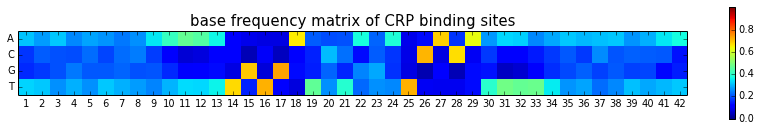#### Entropy function¶

We want to know how conserved the individual positions are. We start by calculating the entropy. The entropy H of nucleotide variability at each site i is defined by, $$H_i=-\sum_{n=\{A,C,T,G\}} p_i(n) \log_2 p_i(n)$$ From the formula, we see that this calculation involves a dot product between the rows of the probability array and the rows of a log probability array. We have to be remember that $0 \log_2 0 = 0$, if any of the probabilities are zero. This is important since $\log_20=-\infty$ and computers don't like dealing with infinities. A simple way to deal with this is to remove all the zero probabilities in the above entropy summation formula.

Let's use this opportunity to go over a simple example of how to define a new function in Python.

In :
# defining a new function 'entropy_calc' which takes as input a 1D array p
def entropy_calc(p):
p=p[p!=0] # modify p to include only those elements that are not equal to 0
return np.dot(-p,log2(p)) # the function returns the entropy result


Let's plug in a few probability distributions into our entropy function to make sure it spits out the right answer

In :
# synthetic probability distributions
dist1=np.array([1/4,1/4,1/4,1/4])
dist2=np.array([1/8,1/8,1/4,1/2])
dist3=np.array([0,0,1/2,1/2])
dist4=np.array([0,0,0,1])

# evaluate the entropies
print entropy_calc(dist1)
print entropy_calc(dist2)
print entropy_calc(dist3)
print entropy_calc(dist4)

2.0
1.75
1.0
-0


Returning to our DNA sequence probabilities, we can evaluate the entropy at each nucleotide position by the following simple command

In :
# loop through every row of the 'prob' array and evaluate the entropy
DNA_entropy=np.array([entropy_calc(t) for t in prob])


This creates a new 1-dimensional NumPy array called 'DNA_entropy'.

In :
print DNA_entropy

[ 1.93058651  1.96552364  1.97261799  1.98543983  1.98914028  1.96977195
1.99007327  1.99055291  1.95423939  1.83532602  1.67642971  1.72522979
1.83103394  1.41673422  1.32210514  1.28901589  1.22955655  1.45681499
1.8307097   1.98013624  1.91557164  1.90779924  1.98933969  1.91490193
1.29159019  1.27154435  1.37822325  1.37352383  1.53361903  1.89163949
1.64024155  1.69155439  1.78068749  1.93158424  1.9718647   1.97030341
1.97617255  1.9764238   1.9741195   1.97573143  1.90948341  1.87516361]


We see that most entropy values are fairly close to the maximum of two bits, representing a great deal of base variability. Let's plot the results.

In :
# number of nucleotide positions
num_pos=len(DNA_entropy)

# set the size of the figure
plt.figure(figsize=(12,4))

# plot a bar chart
plt.bar(np.arange(num_pos),DNA_entropy,color='green')

# axes labels
plt.xlabel('position',fontsize=15)
plt.ylabel('entropy (bits)',fontsize=15)

# limit the x axis range to just to the total number of nucleotide positions
plt.xlim(0,num_pos)

# place the x axis ticks and labels to be centered at each bar
plt.xticks(arange(num_pos)+0.5,arange(1,num_pos+1),fontsize=10)

# show the figure
plt.show()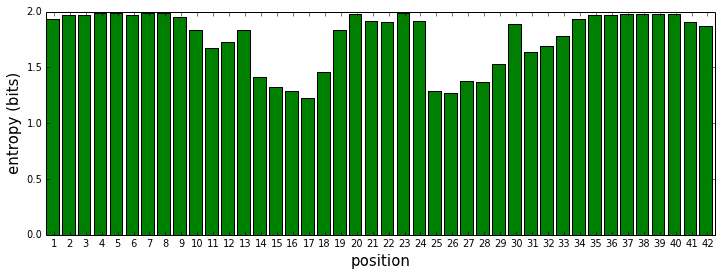What we really want to calculate is the sequence conservation, R. This is defined to be the total possible entropy at each site minus the observed entropy: $$\begin{array} RR &=& H_{max}-H_{obs} \\ &=& \log_2(\rm{no. of states})-\left( - \sum_{n=\{A,C,T,G\}} p(n) \log_2 p(n) \right) \\ &=& 2 + \sum_{n=\{A,C,T,G\}} p(n) \log_2 p(n) \end{array}$$

In :
# conservation score at each position
R=2-DNA_entropy

# set the size of the figure
plt.figure(figsize=(12,4))

# plot a bar chart
plt.bar(np.arange(num_pos),R,color='green')

# axes labels
plt.xlabel('position',fontsize=20)
plt.ylabel('bits',fontsize=20)

# limit the x axis range to just to the total number of nucleotide positions
plt.xlim(0,num_pos)
plt.ylim(0,2)

# place the x axis ticks and labels to be centered at each bar
plt.xticks(arange(num_pos)+0.5,arange(1,num_pos+1),fontsize=10,rotation=0)

# set the title
plt.title('Sequence Conservation of Binding Site', fontsize=20)

# show the figure
plt.show()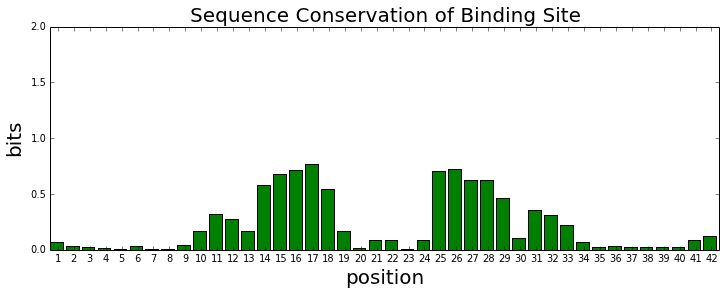### 3. Random sampling, correlations and statistical tests¶

#### Gaussian distribution¶

A frequent task in scientific programming is to analyze random samples from a known distribution. A commonly used distribution is the gaussian (normal distribution)

$$p(x)=\frac{1}{\sqrt{2 \pi \sigma^2}} \exp \left( -\frac{(x-\mu)^2}{2 \sigma^2} \right)$$

where $\sigma$ is the standard deviation and $\mu$ is the mean

In :
# number of samples
N_gauss = 5000

# standard deviations
s = 2

# mean
u = 5

# draw random samples from gaussian distribution
samples=np.random.normal(u,s,N_gauss)

In :
samples

Out:
array([  3.59809573,   6.37121742,   4.22467472, ...,   0.51609674,
5.11093013,  10.53749147])

We can plot the data in two different ways:

In :
plt.figure(figsize=(12,5))

# plot histogram
plt.subplot(1,2,1)
plt.hist(samples,bins=20)
plt.title('histogram')

# plot boxplot
plt.subplot(1,2,2)
# the default is to produce a vertical boxplot. Setting vert=False gives a horizontal plot
plt.boxplot(samples,vert=False)
plt.title('boxplot')

plt.show()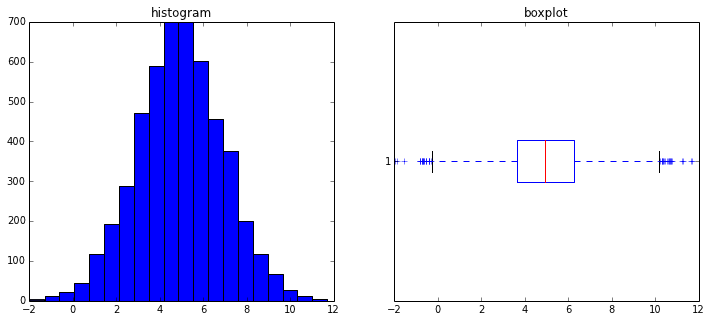We can draw random samples from a two-dimensional Gaussian (normal) distribution centered around the origin, where the $x$ and $y$ variables are independent and therefore uncorrelated,

$$\begin{array} pp(x,y)&=&p(x)p(y) \\ &=&\displaystyle \frac{1}{2 \pi \sigma_x \sigma_y} \exp{\left( -\frac{x^2}{2 \sigma_x^2} - \frac{y^2}{2 \sigma_y^2} \right)} \end{array}$$

In :
plt.figure(figsize=(10,5)) # set the figure size

cm = plt.cm.RdBu # coloring scheme for contour plots

N_2dgauss=100 # number of datapoints

sx=7 # standard deviation in the x direction
sy=2 # standard deviation in the y direction

x=np.random.normal(0,sx,N_2dgauss) # random samples from gaussian in x-direction
y=np.random.normal(0,sy,N_2dgauss) # random samples from gaussian in y-direction

axs=max(abs(x))*1.2 # axes plotting range

t=linspace(-axs,axs,1000) # range of mesh points for contour plot
cx,cy=np.meshgrid(t,t) # mesh array
z=(1/(2*pi*sx*sy))*exp(-((cx*cx)/(2*sx*sx))-((cy*cy)/(2*sy*sy))) # actual 2d gaussian

plt.xlim([-axs,axs])
plt.ylim([-axs,axs])

# plots contour of 2d gaussian
plt.subplot(1,2,1,aspect='equal') # setting axes scales to be equal
contour(t,t,z,linspace(0.0000001,1/(2*pi*sx*sy),20),cmap=cm)
plt.grid()
plt.title('contour map of 2d gaussian distribution\n $\sigma_x=$ %d, $\sigma_y=$ %d' % (sx,sy))
plt.xlabel('x')
plt.ylabel('y')

# plots the random samples on top of contour plot
plt.subplot(1,2,2,aspect='equal')
contour(t,t,z,linspace(0.0000001,1/(2*pi*sx*sy),20),cmap=cm)
plt.plot(x,y,'bo',markersize=5)
plt.grid()
plt.title('%d random samples from the 2d gaussian\n distribution' % N_2dgauss)
plt.xlabel('x')
plt.ylabel('y')

plt.show()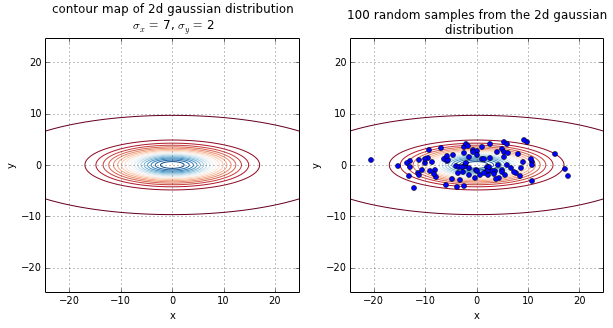#### Covariance and Correlation¶

We can calculate the covariance and correlation of the above data. As a reminder, the covariance matrix of two random variables $x$ and $y$ with associated distributions $p(x)$ and $p(y)$ is

$${\rm{covariance}}= \left( \begin{array}{cc} \langle (x - \langle x \rangle )^2 \rangle & \langle (x - \langle x \rangle)(y - \langle y \rangle) \rangle \\ \langle (y - \langle y \rangle)(x - \langle x \rangle) \rangle & \langle (y - \langle y \rangle )^2 \rangle \end{array} \right)$$

where the $\left< \right>$ brackets denotes the expectation or averaging with respect to the probability distributions (conventional physics notation). The covariance matrix is symmetric and the diagonal elements are the variances in the $x$ and $y$ directions. In practise, with real data, you don't have access to the underlying distributions $p(x)$ and $p(y)$ and so the means and variances have to be estimated from the finite number of samples.

In :
# covariance matrix of the sample
np.cov(x,y)

Out:
array([[ 55.66090714,   1.64811708],
[  1.64811708,   4.63377961]])

The diagonal elements of the sample covariance matrix should be close to the variances (standard deviation squared) of the original gaussian distributions from which the data was sampled from. The more samples the better the agreement.

The correlation matrix is a normalized covariance matrix. The off-diagonal element is the correlation coefficient between x and y, and ranges from -1 to 1, $${\rm{correlation}}= \left( \begin{array}{cc} 1 & \frac{\langle (x- \langle x \rangle)(y-\langle y \rangle) \rangle } {\sqrt{\langle ( x - \langle x \rangle)^2 \rangle } \sqrt{\langle ( y - \langle y \rangle)^2 \rangle } } \\ \frac{\langle (y- \langle y \rangle)(x-\langle x \rangle) \rangle } {\sqrt{\langle ( y - \langle y \rangle)^2 \rangle } \sqrt{\langle ( x - \langle x \rangle)^2 \rangle } } & 1 \end{array} \right)$$

In :
# correlation matrix of the sample
np.corrcoef(x,y)

Out:
array([[ 1.        ,  0.10262312],
[ 0.10262312,  1.        ]])

Note that the correlation between $x$ and $y$ variables is not exactly equal to zero even though the variables are sampled from independent distributions. This illustrates an important point: when the number of samples is low, artificial correlations become likely due to statistical fluctutations.

Another key thing to note is that the correlation between $x$ and $y$ variables, also known as Pearson correlation coefficient, is only sensitive to linear relationships. The following picture from Wikipedia shows the Pearson correlation coefficient for several sets of ($x$,$y$) points. Note that the coefficient does a bad job of detecting non-linear relationships (last row).#### Neural spike rate exercise¶

Let's imagine we have observed firing rates of a neuron when presented with two different stimuli, A and B. We will assume the observations are random samples from two gaussian distributions with differing means.

In :
# number of samples
N=1000

# standard deviation, the same for both stimuli
s=2

# means corresponding to differing stimuli
u_A=8
u_B=12

# observed firing rates
samples_A=np.random.normal(u_A,s,N)
samples_B=np.random.normal(u_B,s,N)

In :
bins=arange(0,20,1) # bin boundaries for both histograms
plt.hist(samples_A,bins,alpha=0.3) # the alpha parameter adjusts the transparency of the colours
plt.hist(samples_B,bins,alpha=0.3,)
plt.legend(('A','B'))
plt.xlabel('firing rates',fontsize=15)
plt.title('histogram of neural firing rates',fontsize=15)
plt.show()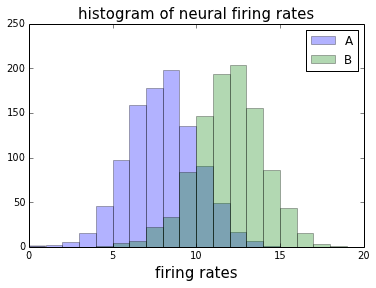If we are given a single firing rate, how do we decide which stimulus was presented? A sensible way would be to impose a threshold at rate=10. However, classification errors are unavoidable, when the neural responses do not separate out cleanly.

Using this strategy, what fraction of the firing rates stemming from stimulus A would the neuron misclassify as deriving from stimulus B?

In :
# threshold of our binary classifier
threshold=10

# misclassified fraction
len(samples_A[samples_A > threshold])/N

Out:
0.164

If we label stimulus B as positive and stimulus A as negative then the above calculation represents the false positive rate in our binary classifier. The true positive rate is then

In :
len(samples_B[samples_B > threshold])/N

Out:
0.849

Clearly, we can carry out a better job at classifying if the distributions were more widely separated by having smaller variability or bigger differences in their means.

Sometimes in data analysis we want to know if two sets of samples have the same mean or are significantly different. There is an example of a statistical test and there are many such tests implemented in SciPy. As an example, we can carry out a traditional t-test for the null hypothesis that the two independent samples from above have identical means

In :
# load the stats module from the SciPy package
import scipy.stats as st

# perform the two-sided t-test, asumming populations have identical variances
t, pval = st.stats.ttest_ind(samples_A,samples_B)

In :
# t-statistic
print t

-44.4406842366

In :
# p-value
print pval

1.5183837236e-300


Statisticians love p-values and will make conclusions about hypotheses based on them. Do not get me started!

### Curve Fitting¶

A frequent task in science is to fit a curve to data, and guess the underyling generative model. Let's make up some fake noisy data of the form $y=x^3 + \eta$, where $\eta$ is noise drawn from a gaussian (normal) distribution.

In :
# number of data points
N=20

# N equalled spaced x values, from -20 to 20
x=np.linspace(-20,20,N)

# noise term: N samples from a gaussian distribution with mean 0 and standard deviation 1000
noise=np.random.normal(0,1000,N)

# y values
y=x**3+noise


Let's plot our fake noisy data.

In :
plt.plot(x,y,'ro') # 'r' indicates red, 'o' indicates a small circle
plt.title('noisy data')
plt.xlabel('x')
plt.ylabel('y')
plt.show()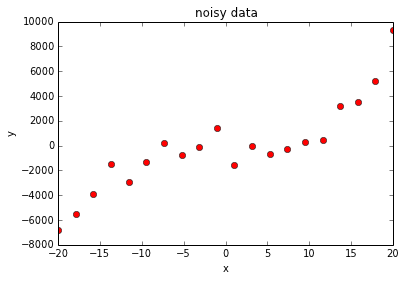Now we will try to fit polynomials of various orders using the Numpy "polyfit" function.

In :
# straight line fit. The "fit1" array consists of the coefficients of the best linear fit
fit1=np.polyfit(x,y,1)

# 3rd order polynomial fit
fit3=np.polyfit(x,y,3)

# 19th order polynomial fit
fit19=np.polyfit(x,y,19)

# create functions from the fits
y_1=np.poly1d(fit1)
y_3=np.poly1d(fit3)
y_19=np.poly1d(fit19)

print('linear fit: y_1=(%.2f)x+(%.2f)' % (fit1,fit1))
print('3rd order fit: y_3=(%.2f)x^3 + (%.2f)x^2 + (%.2f)x + (%.2f)' % (fit3,fit3,fit3,fit3))

linear fit: y_1=(252.25)x+(-77.40)
3rd order fit: y_3=(1.07)x^3 + (2.63)x^2 + (-31.45)x + (-464.81)

In :
plt.plot(x,y,'ro')
plt.plot(x,y_1(x))
plt.plot(x,y_3(x))
plt.plot(x,y_19(x))

# add a legend to the right of the plot
legend_text=('data','linear fit: $y=mx+c$ ','3rd order polynomial','19th order polynomial')
plt.legend(legend_text, loc='center left', bbox_to_anchor=(1,0.5))

plt.show()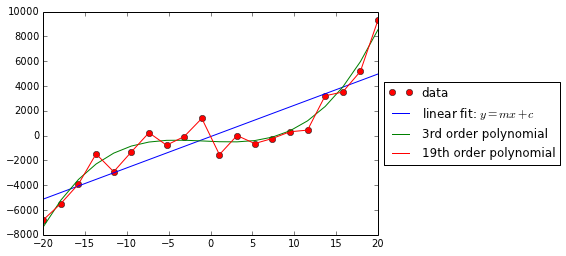The high order (19th) fit clearly tracks the data better. However, the 19th order polynomial in fact overfits the data since it will perform poorly on new data sampled from the original noisy curve.